Home

### Equations and systems with randomness (34Fxx)

• Article
• ##### Corrigendum: On the use of a discrete form of the Itô formula in the article ‘Almost sure asymptotic stability analysis of the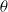$\theta$ -Maruyama method applied to a test system with stabilising and destabilising stochastic perturbations’
• LMS Journal of Computation and Mathematics, Volume 16

### Differential equations in the complex domain (34Mxx)

• Article
• ##### RIGID LOCAL SYSTEMS ON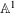$\mathbb{A}^{1}$ WITH FINITE MONODROMY
• Mathematika, Volume 64, Issue 3

• Article
• ##### ON ALGEBRAIC DIFFERENTIAL EQUATIONS FOR THE GAMMA FUNCTION AND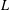$L$ -FUNCTIONS IN THE EXTENDED SELBERG CLASS
• Bulletin of the Australian Mathematical Society, Volume 96, Issue 1
• Article
• ##### HYPERTRANSCENDENCE OF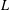$L$ -FUNCTIONS FOR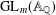$\text{GL}_{m}(\mathbb{A}_{\mathbb{Q}})$
• Bulletin of the Australian Mathematical Society, Volume 93, Issue 3